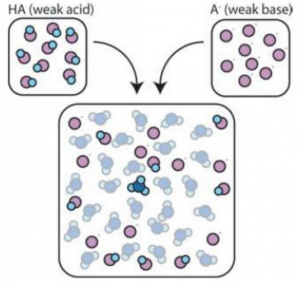# Acids and Bases (2 of 2)

Part 1 of Acids and Bases is located here.

## Dissociation Constant

At equilibrium, the rate of dissociation of the product `AB` is equal to the rate of associate of the reactant `A` and `B`

∴ at equilibrium:

Kd = [A] [B] / [AB]

Which is derived from an example such as: `AB ↔ A + B @ Equilibrium`

## pH Indicators

pH indicators work because of the dissociation of the H+ ions in the indicator itself.

 Common Indicators Range Phenolphthalein 8 – 10 Methyl Red 4.5 – 6 Bromothymol 6 – 7.6

## Buffer Solutions

Buffer solutions resist changes in pH.The buffer solution absorbs the proton

Example:

HA + H2O ↔ H3O+ + A

Ka = [H3O+] [A] / [HA]

-log(Ka) = -log([H3O+] ([A] / [HA]))

-log(Ka) = -log([H3O+]) – log([A] / [HA])

pKa = pH – log([A] / [HA])

pH = pKa + log([A] / [HA])